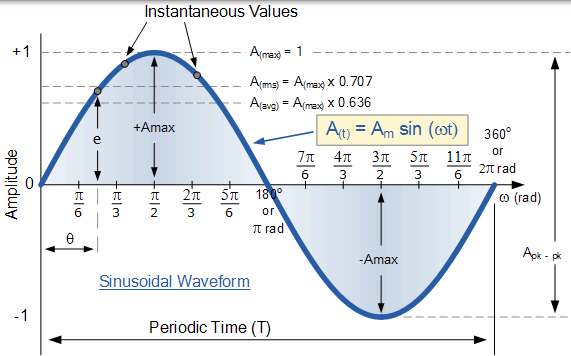# 正弦波形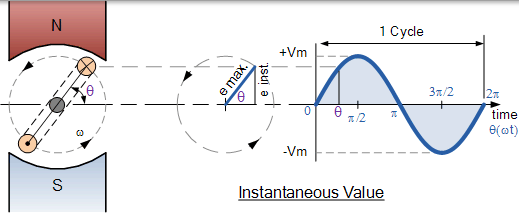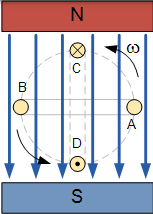### 基本单线圈交流发电机• 速度 - 线圈在磁场内旋转的速度。
• 强度 - 磁场的强度。
• 长度 - 通过磁场的线圈或导体的长度。

$$f = \frac {NP}{60} \mathrm{Hz}$$

## 瞬时电压

### 磁场内线圈的位移$$V _ { i } = V _ { \max } \times \sin \theta$$

### 正弦波形构造

e =Vmax * sinθ 0 70.71 100 70.71 0 -70.71 -100 -70.71 -0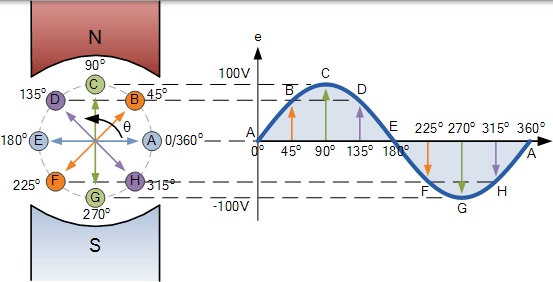## 弧度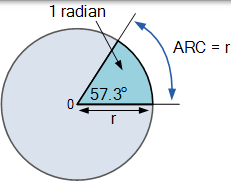$$\begin{array} { c } { 2 \pi \mathrm { rads } = 360 ^ { \circ } } \ { \therefore 1 \mathrm { rad } = 57.3 ^ { \circ } } \end{array}$$

### 度和弧度之间的关系

$$\begin{array} { l } { \text { Radians } = \left( \frac { \pi } { 180 ^ { \circ } } \right) \times \text { degrees } } \\ { \text { Degrees } = \left( \frac { 180 ^ { \circ } } { \pi } \right) \times \text { radians } } \end{array}$$

$$30 ^ { \circ } \rightarrow \text { Radians } = \frac { \pi } { 180 ^ { \circ } } \left( 30 ^ { \circ } \right) = \frac { \pi } { 6 } \mathrm { rad }$$

$$90 ^ { \circ } \rightarrow \text { Radians } = \frac { \pi } { 180 ^ { \circ } } \left( 90 ^ { \circ } \right) = \frac { \pi } { 2 } \mathrm { rad }$$

$$\frac { 5 \pi } { 4 } \mathrm { rad } \rightarrow \text { Degrees } = \frac { 180 ^ { \circ } } { \pi } \left( \frac { 5 \pi } { 4 } \right) = 225 ^ { \circ }$$

$$\frac { 3 \pi } { 2 } \mathrm { rad } \rightarrow \text { Degrees } = \frac { 180 ^ { \circ } } { \pi } \left( \frac { 3 \pi } { 2 } \right) = 270 ^ { \circ }$$

### 度和弧度之间的关系

0o 0 135o 3π 4 270o 3π 2
30o π 6 150o 5π 6 300o 5π 3
45o π 4 180o π 315o 7π 4
60o π 3 210o 7π 6 330o 11π 6
90o π 2 225o 5π 4 360o
120o 2π 3 240o 4π 3

### 正弦波形的角速度

$$\omega = 2 \pi f ( \text { rad } / \text { sec } )$$

$$\omega = \frac { 2 \pi } { \mathrm { T } } ( \mathrm { rad } / \mathrm { sec } )$$

## 正弦波形示例 No1

$${ \mathrm { V } _ { ( \mathrm { ms } ) } = 0.707 \times \mathrm { maximum } \text { peak value } } \\ { \mathrm { V } _ { ( \mathrm { ms } ) } = 0.707 \times 169.8 = 120 \mathrm { volts } }$$

$$\text { Frequency } ( f ) = \frac { 377 } { 2 \pi } = 60 \mathrm { Hz }$$

6ms 时间后的瞬时电压 Vi 值如下：

$$\mathrm { V } _ { ( \mathrm { i } ) } = \mathrm { V } _ { \mathrm { m } } \sin \left( \omega \mathrm { t } \right) \\ { \mathrm { V } _ { ( \mathrm { i } ) } = 169.8 \sin ( 377 \times 0.006 ) } \\ \mathrm { V } _ { ( \mathrm { i } ) } = 169.8 \sin ( 2.262 \mathrm { rads } ) \\ 2.262 \mathrm { rads } \times 57.3 ^ { \circ } = 129.6 ^ { \circ } \\ V _ { ( 1 ) } = 169.8 \sin \left( 129.6 ^ { \circ } \right) = 169.8 \times 0.771 \\ \therefore V _ { ( 1 ) } = 130.8 \text { volts peak }$$

$$\begin{array} { c } { \text { Degrees } = \left( \frac { 180 ^ { \circ } } { \pi } \right) \times \text { radians } } \\ { \therefore \frac { 180 ^ { \circ } } { \pi } \times 2.262 = 57.3 ^ { \circ } \times 2.262 = 129.6 ^ { \circ } } \end{array}$$

## 正弦波形

### 正弦波形